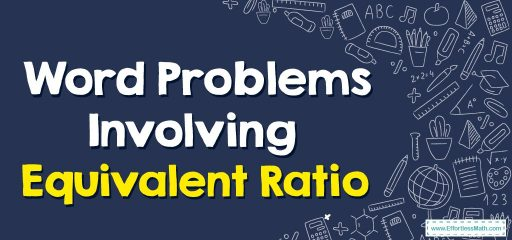# Word Problems Involving Equivalent Ratio

An equivalent ratio is a ratio that has the same relationship between numbers as another ratio, but the numbers themselves may be different.For example, the ratio 2:4 is equivalent to the ratio 4:8 because in both cases, the relationship between the numbers is the same (1:2).

## Step-by-step guide to solving equivalent ratios word problems

To solve the equivalent ratio’s word problems, follow these steps:
Step 1: Find the ratio given in the word problem.
Step 2: Find the given value of one of the two values involved in the ratio
Step 3: Divide the given value of step 2 by the corresponding value in the ratio from step 1.
Step 4: Multiply both numbers in the original ratio by the number found in step 3 to construct the equivalent ratio.

### Word Problems Involving Equivalent Ratio – Examples 1

If a box contains pink and green marbles in the ratio of 3:8 pink to green, how many pink marbles are there if 40 green marbles are in the box?
Solution:
Step 1:
Write the ratios. $$\frac{3}{8}=\frac{x}{40}$$
Step 2: Use cross multiplication. $$3×40=8×x⇒120=8x$$
Step 3: Divide. $$x=\frac{120}{8}=15$$

### Word Problems Involving Equivalent Ratio – Examples 2

Are these ratios equivalent? 10 books for 15 shelves, and 20 books for 25 shelves.
Solution:
Step 1: Write the ratios. $$\frac{10}{15}, \frac{20}{25}$$
Step 2: Use cross multiplication. $$10×25=20×15⇒250≠300$$
So these ratios are not equivalent.

### What people say about "Word Problems Involving Equivalent Ratio - Effortless Math: We Help Students Learn to LOVE Mathematics"?

No one replied yet.

X
30% OFF

Limited time only!

Save Over 30%

SAVE $5 It was$16.99 now it is \$11.99